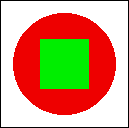### Quiz Questions For Chapter 6

THIS PAGE CONTAINS A SAMPLE quiz on material from Chapter 6 of this on-line Java textbook. You should be able to answer these questions after studying that chapter. Sample answers to all the quiz questions can be found here.

Question 1: Programs written for a graphical user interface have to deal with "events." Explain what is meant by the term event. Give at least two different examples of events, and discuss how a program might respond to those events.

Question 2: What is an event loop?

Question 3: Explain carefully what the repaint() method does.

Question 4: What is HTML?

Question 5: Draw the picture that will be produced by the following paint() method:

```             public static void paint(Graphics g) {
for (int i=10; i <= 210; i = i + 50)
for (int j = 10; j <= 210; j = j + 50)
g.drawLine(i,10,j,60);
}
```

Question 6: Suppose you would like an applet that displays a green square inside a red circle, as illustrated. Write a paint() method that will draw the image.Question 7: Suppose that you are writing an applet, and you want the applet to respond in some way when the user clicks the mouse on the applet. What are the four things you need to remember to put into the source code of your applet?

Question 8: Java has a standard class called MouseEvent. What is the purpose of this class? What does an object of type MouseEvent do?

Question 9: Explain what is meant by input focus. How is the input focus managed in a Java GUI program?

Question 10: Java has a standard class called JPanel. Discuss two ways in which JPanels can be used.

[ Answers | Chapter Index | Main Index ]